# Output Devices¶

Group Assignment For this week the group assignment is to Measure the power consumption of an output device

## Power calculation¶

Electric power is the rate, per unit time, at which electrical energy is transferred by an electric circuit. so power has the units of joules/second, which is called the watt. Electric power is usually produced by electric generators, but can also be supplied by sources such as electric batteries.

Electrical power is the product of voltage and current. To calculate the power we need to Record the circuit’s voltage. A circuit’s voltage is analogous to the force applied in a mechanical movement. The voltage tells you how much electrical charge is being passed through a circuit. And we need to Record the circuit’s current. The current of an electrical circuit is analogous to the velocity applied in a mechanical movement. The current tells you how fast the charge is being passed through the circuit.

When we have the voltage and current, we can calculate power.

Power = Current X Voltage

## DC motor¶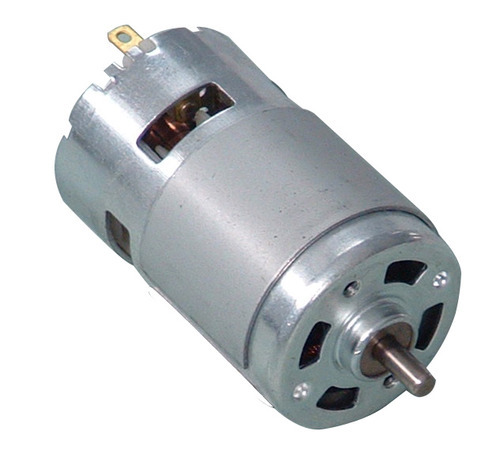A DC motor is any of a class of rotary electrical motors that converts direct current electrical energy into mechanical energy. The most common types rely on the forces produced by magnetic fields.

The DC motor used is a Compact DC Gear Box Motor with a Rated Voltage of DC 12V. The listed Rated Current is 0.3A.

### start power¶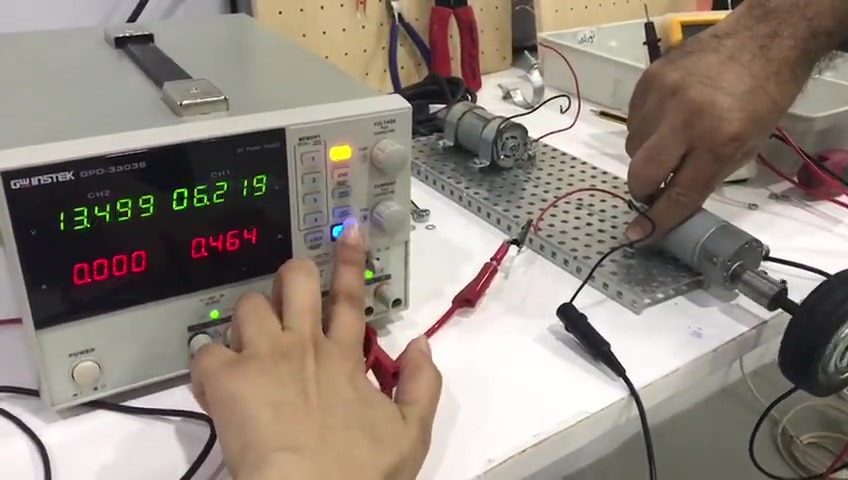P = 6.219V * 0.464A = 2.88w

### normal running power¶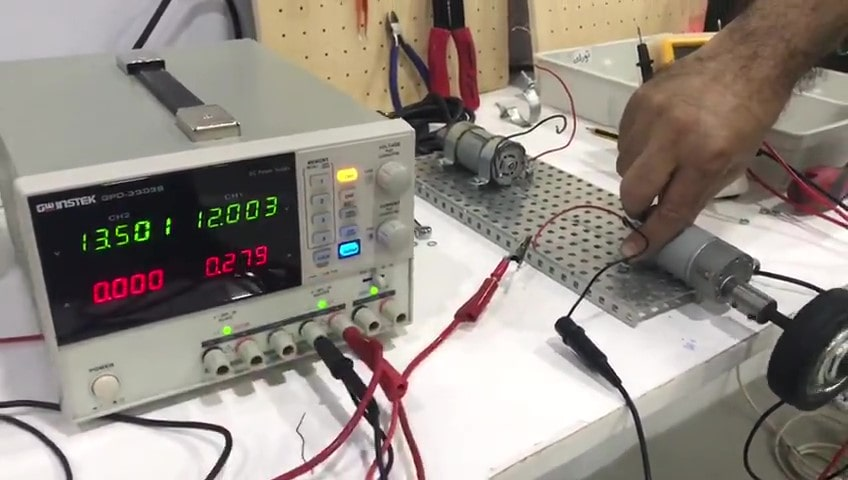P = 12V * 0.279A = 3.348w

### stalled power¶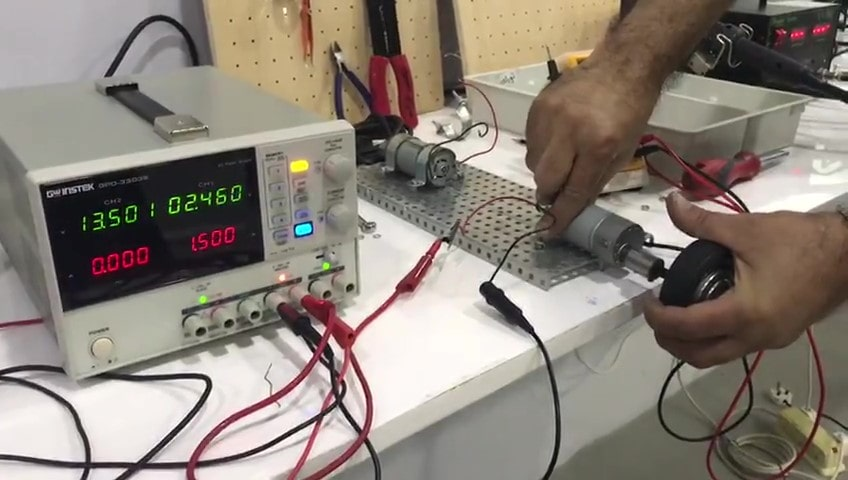P = 2.46V * 1.5A = 3.69w

## Light bulb¶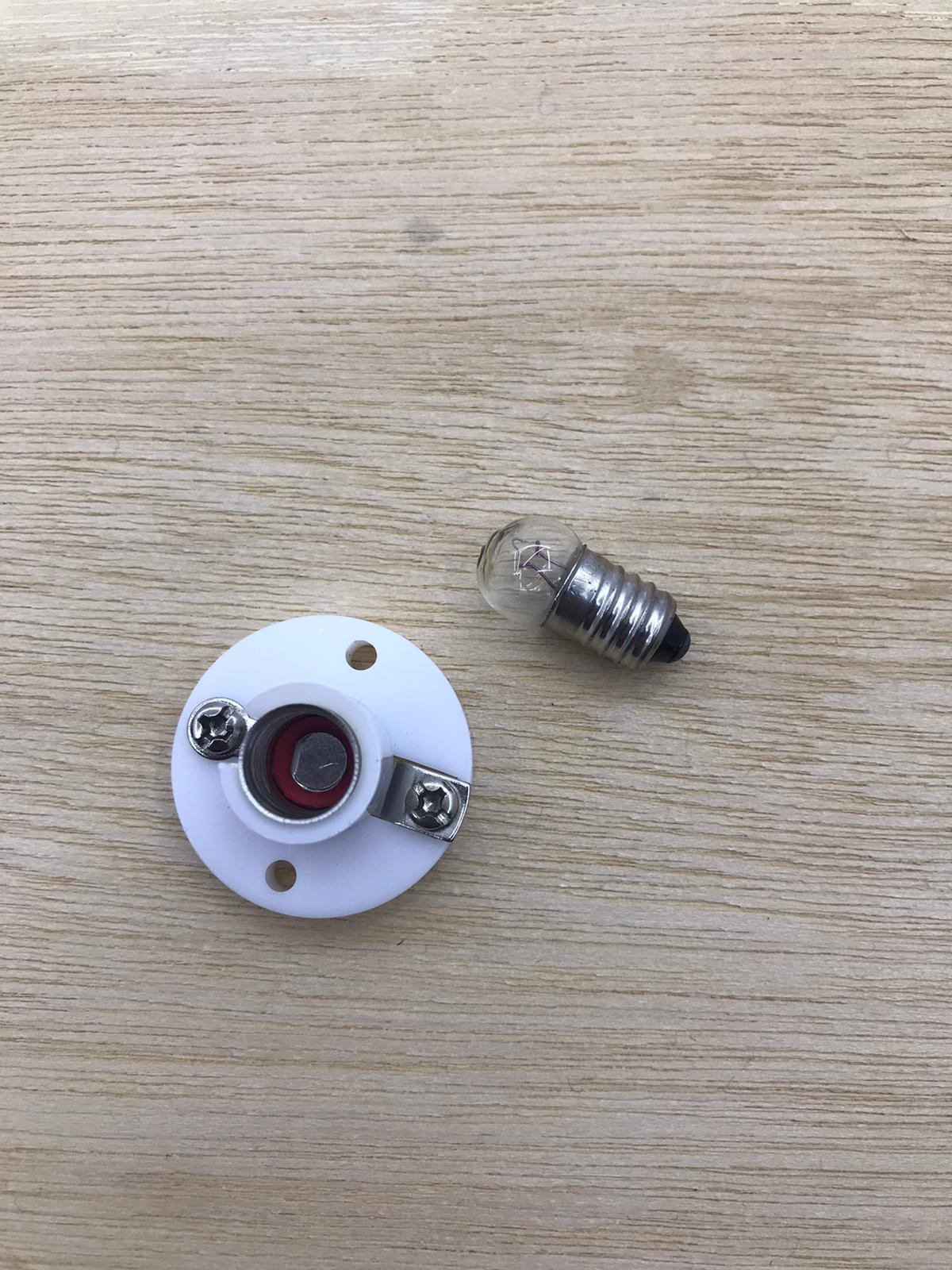An E10 6V Light Bulb Miniature Screw Base Lamp. Those miniature light bulbs have a miniature screw base thats a 10mm diameter and screws into a compatible socket. The listed current is 0.5A.

### bulb power¶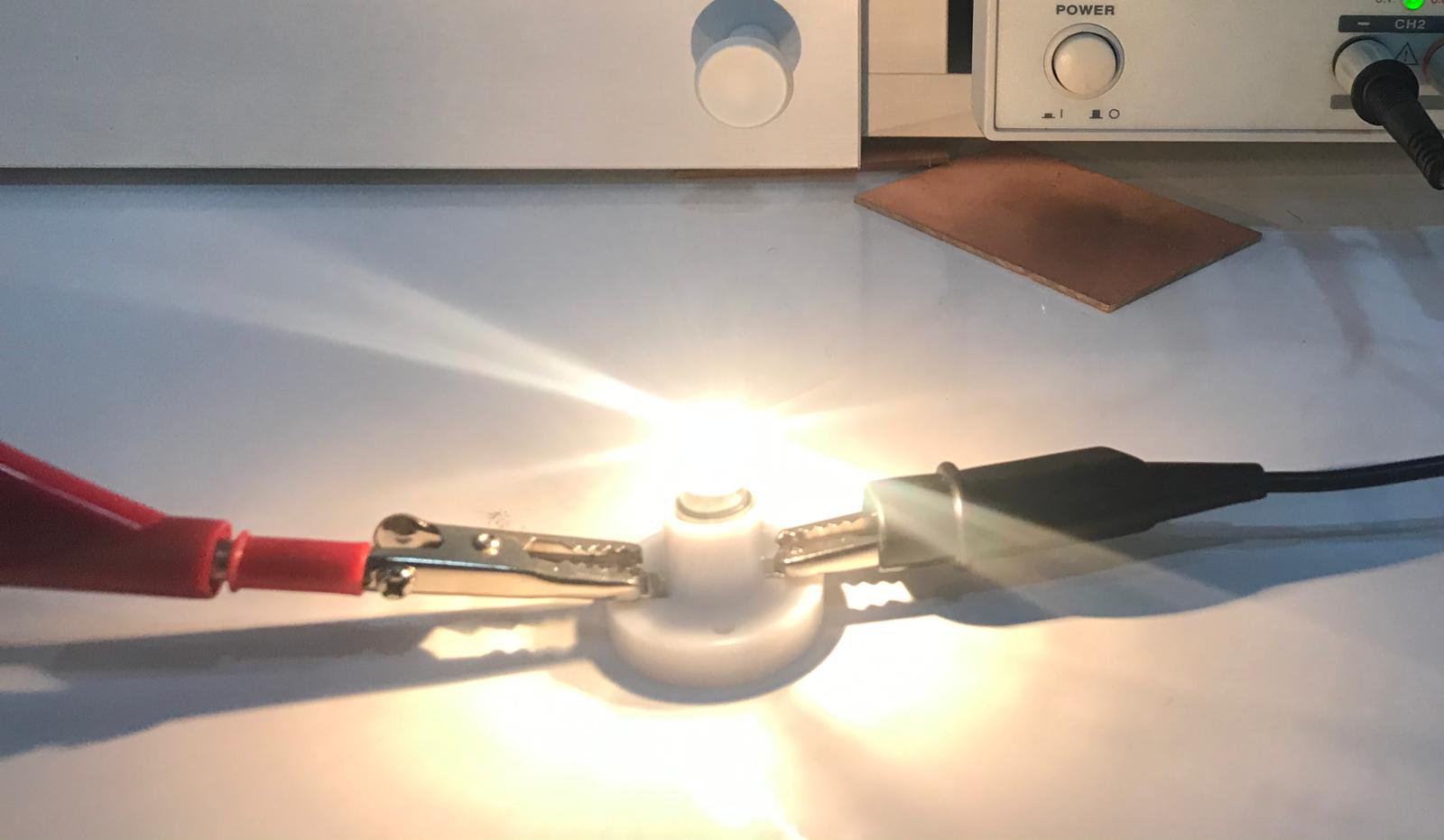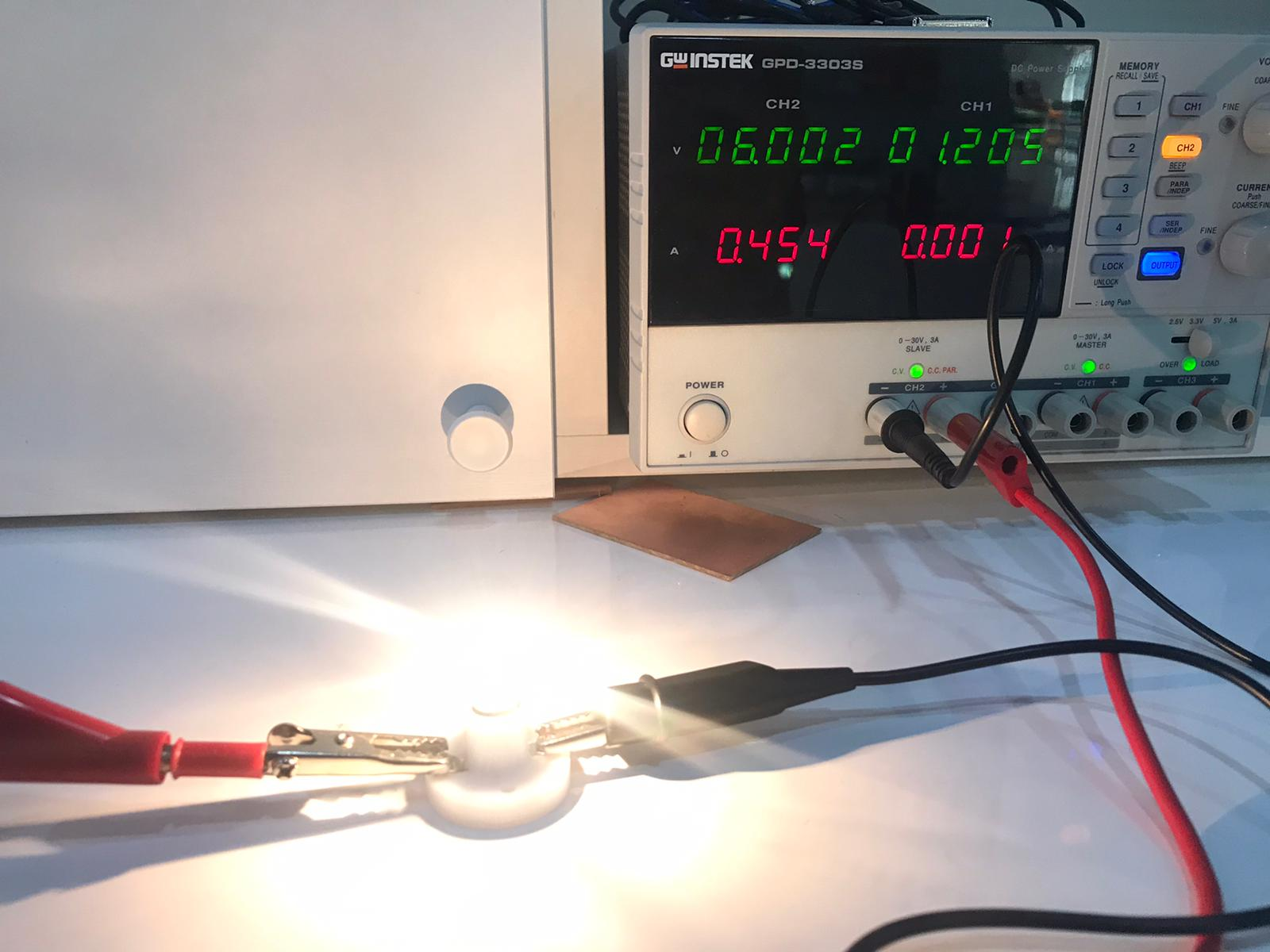p= 6V * 0.454 A = 2.724w